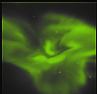Next: Error sensitivity Up: Tomography Previous: Introduction   Contents

# Resolution

This section analyses the resolution of the tomographic model system outlined in the previous section both by singular value decomposition and model tests. In paper I, I tried to apply the standard method of calculating the 3-D resolution for ALIS by singular value decomposition (SVD). Due to the curse of dimensionality the number of voxels had to be reduced from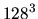toand the number of pixels per image had to be reduced from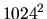to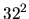thus restricting the possible conclusions about the three-dimensional resolution.

Now with the two-dimensional tomographic model system with imaging stations at 50 km north, 0, 50 and 100 km south, it is possible to apply the SVD analysis with a vertical slice with 128 voxels per side and one-dimensional images with 256 pixels. With the cameras directed towards the centre of the voxel slice, the transfer matrices from the stations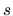have been calculated. Concatenating the station transfer matrices gives a full system transfer matrix:(3.14)

With SVD analysis the resolution in voxel space can be calculated. Given the transfer matrix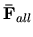from the voxel space down to all images with a singular value decomposition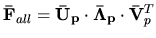, the maximum spatial resolution is given by the resolution matrix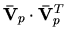( Menke, 1989).

In order to suppress noise in the reconstruction, only the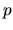singular values larger than 1 in the singular value decomposition ofabove are kept. To illustrate the result of the SVD analysis, we calculate the point spread of three input points at altitudes of 120, 152 and 184 km central in the slice. By doing this we get the variation of the point spread function with altitude.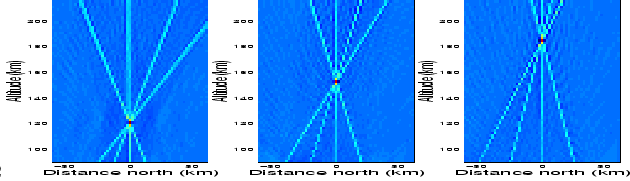As can be seen in Figure 3.5, the point is accurately retrieved in position but there are star-like traces in the directions away from and towards the stations. To quantify the results of the point spread calculations we take vertical cuts through the centres of the point spread functions. This gives the vertical resolution and its variation with altitude.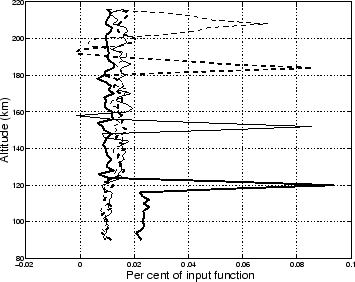As can be seen, the full width at half maximum is 4 km at 120 km and growing to 10 km at 210 km indicating good altitude resolution at all altitudes. The maximum of the point spread function is, however, only 10 % of the input function and the 10 % width in the zenith direction is smeared out at all altitudes. This implies that the individual peaks in the emission distribution are well retrieved, while internal structures at lower intensity levels will be smeared out. To show whether it is possibile to retrieve internal structures in the aurora with a ground-based system it is enlightening to consider a simple volume distribution and set one or more voxels to zero and then calculate the hole retrieval'' in the same way as the point spread was calculated above. In the vertical retrieval of this test, where the simple model is the first column of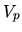that corresponds to the eigenvector with the largest eigenvalue, holes with vertical sizes of 2, 4, 8 and 16 km are used; the result is presented in Figure 3.7. As can be seen, the hole is not retrieved even for the largest hole size.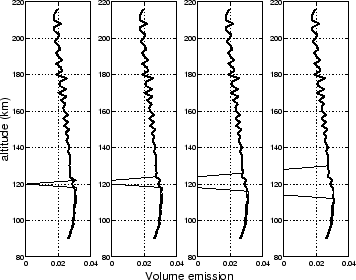Next: Error sensitivity Up: Tomography Previous: Introduction   Contents

copyright Björn Gustavsson 2000-10-24